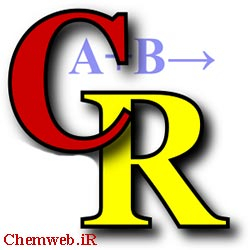September 21, 2021|ChemRate software free download is a tool in chemistry for chemical kinetics and dynamic database Software that enables one to calculate time-dependent and steady-state rate coefficients at different temperatures and pressures. Systems of high complexity involving reversible isomerization, decomposition and thermal and chemical activation can be considered. The density and sum of states are calculated using direct count or Whitten-Rabinovitch algorithms with vibration frequencies and moments of inertia as input parameters. Alternatively, analytical expression with three parameters can be used for k(E). The microscopic rate coefficients k(E) are calculated by using RRKM theory. The collision energy transfer probabilities are taken in the standard form of an “exponential down” model. The time evolution of the population of each the species in the system is described by the master equation.

Key features of ChemRate software:

• Calculates high pressure  rate constants of all  unimolecular reactions on the basis of transition state theory.
• Determines specific rate constants on the basis of RRKM theory.
• Treats multichannel reactions including chemical activation processes under equilibrium and non-equilibrium conditions. Calculates steady state rate constant as well as time-dependent rate constants.
• Carry out calculations with any initial distribution.
• Compute thermofunctions (Cp, Δ, ΔpK, etc.) at any temperature.
• Simple treatment of bimolecular reactions.
• Calculates thermodynamic properties and rate constants from Gaussian file.
• Treats hindered rotors.
• Treats tunneling.
• Multicomponent buffer gas.
• Fit computed results in various ways.
• Compare computed results with experimental data.
• Easy to build time-dependent distribution functions, rate constants, and concentration.